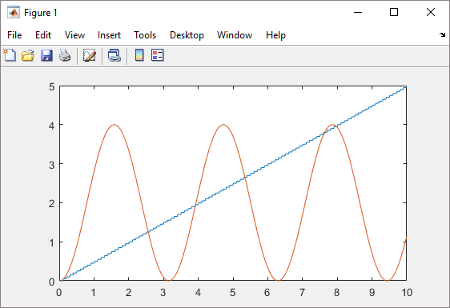## Test Export-Function Model Simulation Using Input Matrix

When function-call sequencing is simple enough to be specified as a model input, simulation using an input matrix is the preferred method for testing an export-function model.

• Create time vectors that specify function-call times.

• Create a matrix that adds input data to the time vectors.

• Run simulation.

To create the model in this example, see Create an Export-Function Model.

### Create Function-Call Inputs and Data Inputs

Create time-vectors indicating when events occur for root-level function-call Inport blocks and data-vectors for root-level data Inport blocks.

1. For function-call Inport blocks `1` and `2`, create column vectors with time steps of `0.1` and `0.01`. In the MATLAB® Command Window, enter

```t1 = [0:0.1:10]'; t2 = [0:0.01:10]';```
• The time vector must be monotonically increasing and of double data type.

• If the sample time for a function-call Inport block is specified, the values in the corresponding time vector must all be integer multiples of the specified value.

• To specify multiple function-calls at a given time step, repeat the time value accordingly. For example, to specify three events at t = 0.1 and 2 events at t = 0.5, list 0.1 three times and 0.5 twice in your time vector, ```t1 = [0.1 0.1 0.1 0.5 0.5]'```.

• To use nonperiodic sample times, set the Inport block Sample time to `-1` and provide a nonuniform time vector, e.g. `t1 = [0, 0.1, 0.2, 0.4, 0.8]`.

2. Create a matrix with time steps and data values for data Inport block `3`.

```sine_data = sin(0:0.01:10)'; d3 = [t2,sine_data];```

The data input can use any supported format as described in Forms of Input Data.

The following table provides additional information for specifying the time vector `t`.

Sample time type for internal Trigger Port block set to:

Root-level Function-Call Inport block with inherited sample time (-1)

Root-level Function-Call Inport block with discrete sample time

`triggered`

Nondecreasing column vector.

The function-call subsystem executes at the times specified by the column vector. The sample times can be periodic or aperiodic.

If you specify an empty matrix (`[]`), the function-call subsystem does not execute.

Nondecreasing column vector. Each element in the column vector must be an integer multiple of the sample time specified by the Inport block.

The function-call subsystem executes at the times specified by the column vector.

Alternatively, specify an empty matrix (`[]`) and the function-call subsystem executes once at every sample time specified by the Inport block.

`periodic`

Configuration not allowed

Empty matrix(`[]`).

The function-call subsystem executes at the times specified by the Inport block calling it.

### Simulate Export-Function Model

Simulate the export-function model to test and observe its behavior before generating code.

1. Import time and data from the MATLAB workspace.

On the Modeling tab, select Model Settings. In the Configuration Parameters dialog box, select the Data Import/Export pane and set the Input parameter to `t1, t2, d3`.

`t1` and `t2` are column vectors containing event times for the function-call Inport blocks `1` and `2`. `d3` is a table of input values versus time for the data Inport block `3`.

2. Run simulation.

3. Plot results. In the MATLAB Command Window, enter.

```plot(yout.time, yout.signals(1).values) hold plot(yout.time, yout.signals(2).values)```4. Change `t1` to provide events every `0.5` seconds (`0.5` is an integer multiple of the sample time of `0.1` specified in Inport block `1`).

`t1 = [0:0.5:10]';`
5. Rerun simulation.After you test your model, you can generate code for the functions. See Generate Code for Export-Function Model.